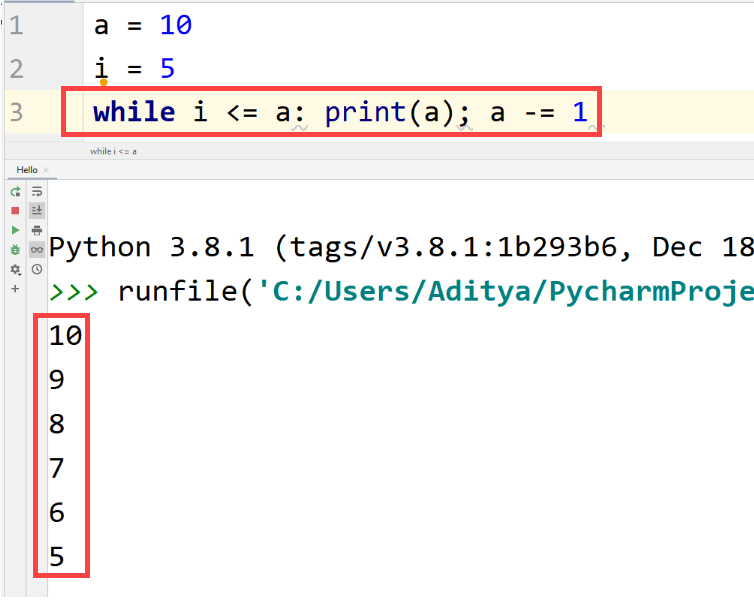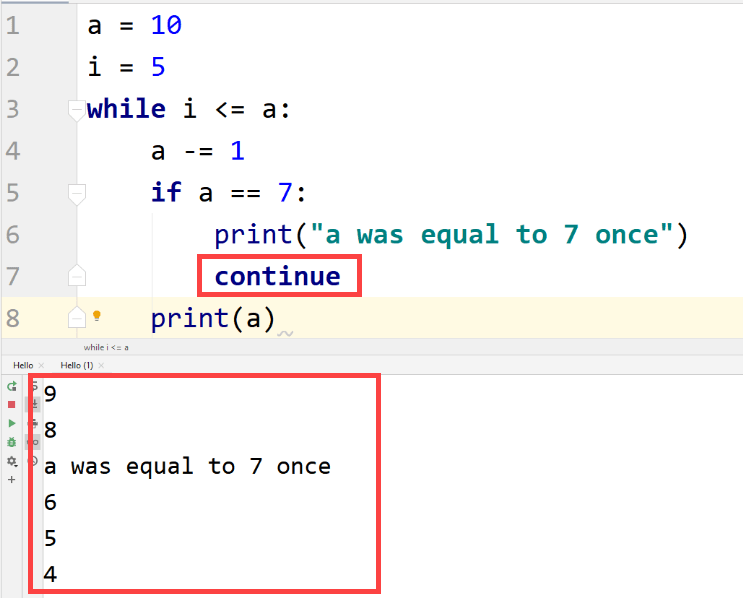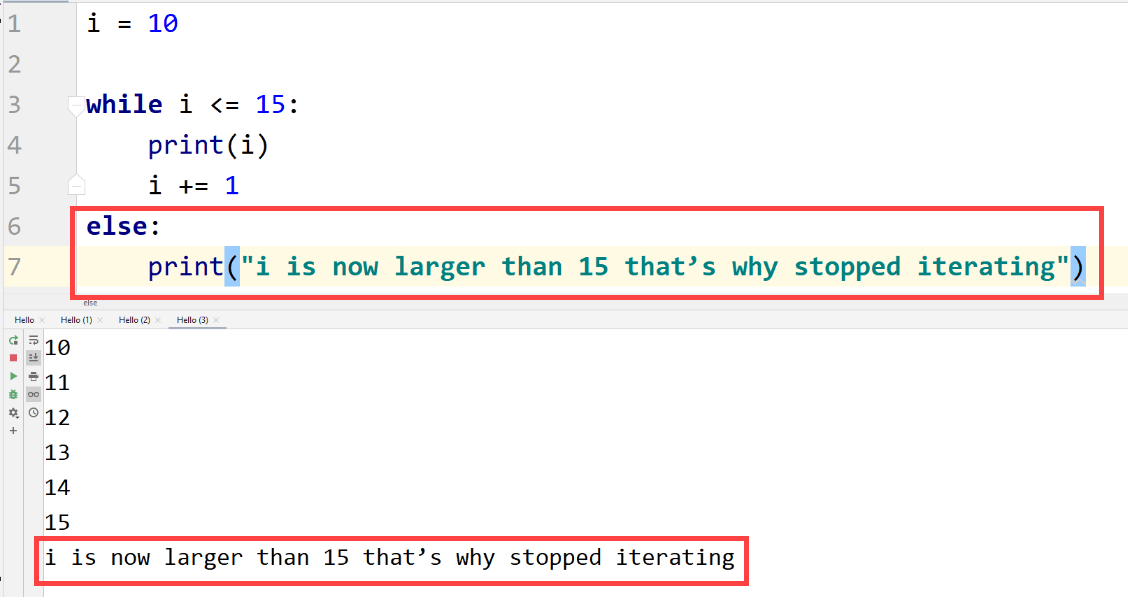# Python While Loop

## Python While Loop

Loops can execute a code statement multiple time. In Python, the while loop can execute a given statement multiple times if the condition provided to the statement is true.

It is used when we don’t know how many times we want to execute/iterate through a piece of code.

Code can be iterated indefinite times using a loop, it means that we have no idea how many times the code will be iterated. The while loop is an indefinite loop, let’s see why by having a look at its syntax.

Syntax
The syntax of the while loop is as follows: –

while condition:
statement(s)

## ExampleLet’s see how the code was executed: –

• We set two variables “a” and “i” where “a” was bigger than “i”.
• Then, by writing this syntax “while i <= a”, we told the computer that you have to execute the code written below that is “print(a)” until the “i” is smaller than or equal to “a”.
• Now we have to change the value of either “i” or “a” to stop the while loop from executing infinite times because “i” will always remain smaller than “a”. This is the reason we wrote “a = a – 1″, now after each iteration, the value of “a” will decrease by 1.

After iterating through the while loop 6 times, the value of “a” becomes equal to 4 which does not satisfy our condition, the while loop breaks itself and the code either ends or moves to next bit of the code.

You can also write while loops in a single line like this –

## ExampleDifferent statements are separated using the semicolon (;).

## continue And break Statements

Sometimes, we don’t want the statement to execute on a given condition or a range of conditions, we can either use continue to continue executing the upcoming iterations and skip the current iterations or break to stop iterating through the loop if a given condition is met.

## continue

Let’s understand it by having an example first –

## Example## break

Similarly, for the break statement:

## ExampleAs you can see in the above code that interpreter didn’t execute after “a” became equal to 7. It is used to “break” or exit the loop when a given condition is true.

## else Statement in while loop

The “else” statement for the while loop is similar to the “else” of if-else statements which stated that “do this if the condition is not satisfied”. Let’s have an example –

## Example## An Infinite while Loop

A while loop can run forever if the condition remains always truth.

## ExampleAs you can see above, the code never stopped looping through a number of given statements and will go till infinity.